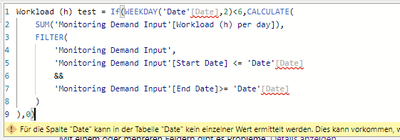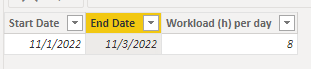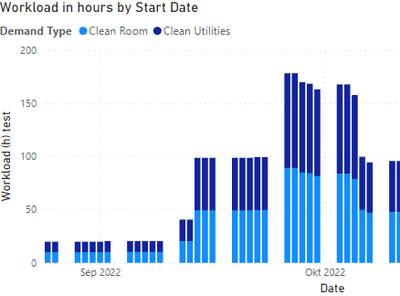cancel
Showing results for
Did you mean:Regular Visitor

## Measure not working with calculated column code due to "no single values"

Hi,

I would like to know how i have to adapt my code for a measure that is working for a calculated column.

There are two tables, one date table and one value table. The value table includes 4 columns of interest:

Project name, Workload (h) per day, Start Date, End date

The calculated column in the date table had to following code to sum up the workload on each day:

SUM('Monitoring Demand Input'[Workload (h) per day]),
FILTER(
'Monitoring Demand Input',
'Monitoring Demand Input'[Start Date] <= 'Date'[Date]
&&
'Monitoring Demand Input'[End Date]>= 'Date'[Date]
)
)

However, this code doesn't work with a measure as the column Date doesn't contain a single/unique value:I need the measure to allow the filtering on the column Project name, which is lost by the calculated column.

Thank you for your help and time!

1 ACCEPTED SOLUTIONCommunity Support

Hi, @GunnZ

Sample data:You can try the following formula.

Measure:

``````Workload (h) test =
IF (
WEEKDAY ( SELECTEDVALUE ( 'Date'[Date] ), 2 ) < 6,
CALCULATE (
SUM ( 'Monitoring Demand Input'[Workload (h) per day] ),
FILTER (
ALL ( 'Monitoring Demand Input' ),
'Monitoring Demand Input'[Start Date] <= SELECTEDVALUE ( 'Date'[Date] )
&& 'Monitoring Demand Input'[End Date] >= SELECTEDVALUE ( 'Date'[Date] )
)
),
0
)``````Best Regards,

Community Support Team _Charlotte

If this post helps, then please consider Accept it as the solution to help the other members find it more quickly.

2 REPLIES 2Community Support

Hi, @GunnZ

Sample data:You can try the following formula.

Measure:

``````Workload (h) test =
IF (
WEEKDAY ( SELECTEDVALUE ( 'Date'[Date] ), 2 ) < 6,
CALCULATE (
SUM ( 'Monitoring Demand Input'[Workload (h) per day] ),
FILTER (
ALL ( 'Monitoring Demand Input' ),
'Monitoring Demand Input'[Start Date] <= SELECTEDVALUE ( 'Date'[Date] )
&& 'Monitoring Demand Input'[End Date] >= SELECTEDVALUE ( 'Date'[Date] )
)
),
0
)``````Best Regards,

Community Support Team _Charlotte

If this post helps, then please consider Accept it as the solution to help the other members find it more quickly.Regular Visitor

Hi Charlotte,

Thank you for the help and showing me SELECTEDVALUE.

However, the filtering is still lost. The sample data has an additonal column called "type".

A slicer doesn't work and if I add the "type" as a legend, it simply duplicates the data:And a follow-up question: to sum up the workload per week, I would create a second measure to sum up the first correct?